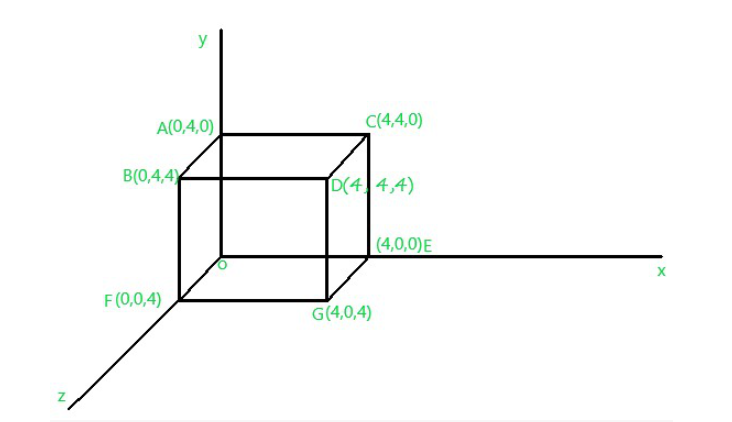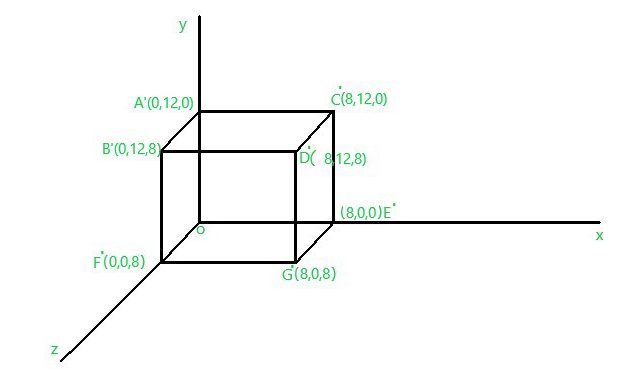Computer Graphics – 3D Scaling Transformation

• Difficulty Level : Medium
• Last Updated : 14 Feb, 2021

Prerequisite: Computer Graphics – 3D Translation Transformation

Scaling Transformation :
It is performed to resize the 3D-object that is the dimension of the object can be scaled(alter) in any of the x, y, z direction through Sx, Sy, Sz scaling factors.

Matrix representation of Scaling transformation Condition :The following kind of sequences occur while performing the scaling transformations on a fixed point –

• The fixed point is translated to the origin.
• The object is scaled.
• The fixed point is translated to its original position.

Let a point in 3D space is P(x, y, z) over which we want to apply Scaling Transformation operation and we are given with Scaling factor [Sx, Sy, Sz] So, the new position of the point after applying Scaling operation would be –Note : If Scaling factor (Sx, Sy, Sz), then, in this case, the 3D object will be Scaled up uniformly in all X, Y, Z direction.

Problem :
Consider the above problem where a cube” OABCDEFG” is given O(0, 0, 0, ), A(0, 4, 0), B(0, 4, 4), C(4, 4, 0), D(4, 4, 4), E(4, 0, 0), F(0, 0, 4), G (4, 0, 4) and we are given with Scaling factor Sx, Sy, Sz. Perform Scaling operation operation over the cube.

Solution :
We are asked to perform the Scaling transformation over the given below 3D object Fig.1:Fig.1

Now, applying the Matrix Scaling transformation condition we get –After performing the Scaling Transformation successfully the Fig.1 will look like as below Fig.2 –Fig.2

My Personal Notes arrow_drop_up